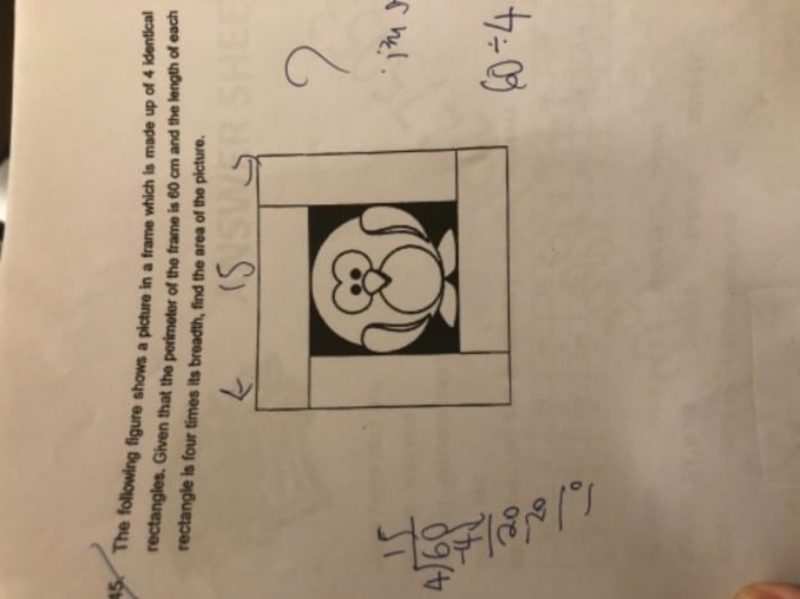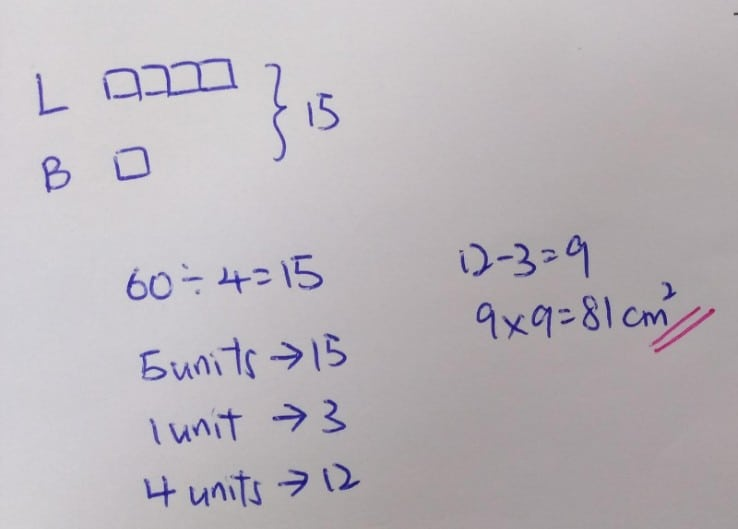# QuestionGiven that the four rectangles are all identical, and based on the layout, the shape of the frame must be a square.

If the perimeter is 60 cm, then each side is 60/4 cm = 15 cm.

Given that the length is 4 times the breadth, each side of the frame is made up of 4 + 1 units (length + 1 breadth).

So, 5 units = 15 cm, so each 1 unit is  15/5 cm = 3 cm.

The picture inside is of length 3 units, as all four sides are enclosed by the frame. Therefore the length of the picture is 3 units x 3 cm = 9 cm.

Area of the picture is therefore 9 x 9 cm = 81 sq cm.

0 Replies 0 Likes